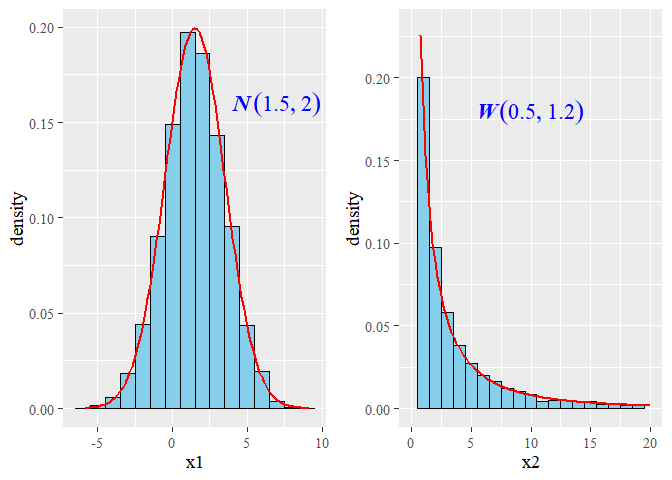# Goodness of fit with large Sample size

## 1. Background

The goodness of fit (GOF) tests frequently fail with real datasets when the sample size goes beyond 100. This issue is critical when working with experimental data where enviromental random noise cannot be prevented. Fortunately, permutation and Monte Carlo approaches for GOF could help to confront the issue. Herein, two examples with simulated data are presented.

## 2. Install and loading the R packages needed for the computation

To run a Monte Carlo (MC) approach for GOF we will install the R package usefr and its dependences from GitHub:

source("https://bioconductor.org/biocLite.R")
biocLite('BiocParallel')
install.packages(c("minpack.lm", "numDeriv", "devtools", "ggplot2", "gridExtra"),
dependencies=TRUE)
devtools::install_git("https://github.com/genomaths/usefr.git")

Next, to load the packages that are needed for this example:

suppressMessages(library(ggplot2))
suppressMessages(library(gridExtra))
suppressMessages(library(usefr))

## 2. Example 1

Let us simulate random samples from a specified Normal and Weibull distributions. To make reproducible this example, we set a seed.

set.seed(1)
x1 = rnorm(10000, mean = 1.5, sd = 2)
x2 = rweibull(10000, shape = 0.5, scale = 1.2)
dt <- data.frame(x1 = x1, x2 = x2)

## 2.1 The histograms and density plots of the given Normal and Weibull distributions

p1 <- ggplot(data = dt, aes(x1)) +
geom_histogram(data = dt, aes(y=..density..), binwidth = 1,
colour = "black", fill = "skyblue", na.rm=TRUE) +
stat_function(fun = dnorm, n = 101, col = "red",
args = list(mean = 1.5, sd = 2), size = 1) +
theme_gray(base_family = "serif", base_size = 14) +
annotate(geom = "text", x = 7, y = 0.16, size = 6,
label = 'bolditalic(N(1.5,2))',
family = "serif", color = "blue", parse = TRUE)

p2 <- ggplot(data = dt, aes(x2)) +
geom_histogram(data = dt, aes(y=..density..), binwidth = 1,
colour = "black", fill = "skyblue", na.rm=TRUE) +
xlim(0,20) + ylim(0,0.23) +
stat_function(fun = dweibull, n = 101, col = "red",
args = list(shape = 0.5, scale = 1.2), size = 1) +
theme_gray(base_family = "serif", base_size = 14) +
annotate(geom = "text", x = 10, y = 0.18, size = 6,
label = 'bolditalic(W(0.5, 1.2))',
family = "serif", color = "blue", parse = TRUE)
grid.arrange(p1, p2, nrow = 1)The bell shape, typical of the normal distribution, is clear. Many people are not familiar with the Weibull distribution. The decay observed in the Weibull distribution presented in the figure is typically found for the for information divergences of DNA methylation levels, in humans and in plants .

## 3. The Monte Carlo GOF test

The test is performed with function mcgoftest from the R package usefr

mcgoftest(x1, cdf = pnorm, pars = c(1.5, 2), num.sampl = 500,
sample.size = 1000, num.cores = 1)
## *** Monte Carlo GoF testing based on Kolmogorov-Smirnov statistic ...##   KS.stat.D      mc_p.value KS.stat.p.value     sample.size       num.sampl ##   0.2534276       0.6007984       0.0000000    1000.0000000     500.0000000 

Kolmogorov-Smirnov (KS) test rejects the Null Hypothesis: KS_p.value $\simeq$ 0. According with KS test there is not enough statistical evidence to support the null hypothesis that the observed values of variable x1 follow a normal distribution with mean 1.5 and standard deviation 2. This looks paradoxical if we take into account that this sample was generated using the theoretical distribution. The fact is that computer algorithms to generate random numbers are not perfect and, so far, the numerical algorithms can only generate pseudo-random numbers, which mostly follow the theoretical distribution. The Monte Carlo sampling approach, however, does not reject the Null Hypothesis: mc_p.value = 0.5908184. However, the failure of KS test would be the result of more deep mathematical-philosophical issues.

The testing for the Weibull distribution yields similar results:

mcgoftest(x2, cdf = pweibull, pars = c(shape = 0.5, scale = 1.2), num.sampl = 500,
sample.size = 1000, num.cores = 1)
## *** Monte Carlo GoF testing based on Kolmogorov-Smirnov statistic ...##      KS.stat.D      mc_p.value KS.stat.p.value     sample.size       num.sampl ##      0.1947641       0.8323353       0.0000000    1000.0000000     500.0000000​

MC KS test does not reject the null hypothesis that variable x comes from Weibull(x|shape = 0.5, scale = 1.2), while the standard Kolmogorov-Smirnov test rejects the Null Hypothesis.

## References

1. Sanchez, Robersy, and Sally A. Mackenzie. 2016. “Information Thermodynamics of Cytosine DNA Methylation.” Edited by Barbara Bardoni. PLOS ONE 11 (3). Public Library of Science: e0150427. doi:10.1371/journal.pone.0150427.